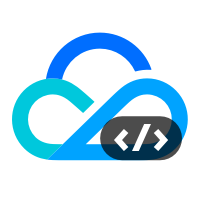# 用云开发数据库实现列表触底自动加载功能丨云开发101

## 微信小程序实现触底自动加载

#### 实现代码

``````Page({
data:{
items:[] // 用于放置数据的数组。
},
// 页面加载完成后，调用此函数
},
onReachBottom:function(){
// 页面滑动触底后，调用此函数
},
// 加载数据所用函数
}
})
``````

``````Page({
data: {
items: [] // 用于放置数据的数组。
},
},
onReachBottom: function () {
},
// 加载数据所用函数
}
})
``````

``````loadData: function () {
let old_data = this.data.items;
const db = wx.cloud.database();
db.collection('items').where({
done: false,
}).skip(old_data.length).get().then(res => {
this.setData({
items:old_data.concat(res.data.data)
})
})
}
``````

``````Page({
data: {
items: []
},
},
onReachBottom: function () {
},
let old_data = this.data.items;
const db = wx.cloud.database();
db.collection('items').where({
done: false,
}).skip(this.data.items.length).get().then(res => {
this.setData({
items:old_data.concat(res.data.data)
})
})
}
})
``````

``````<view wx:for="{{items}}" wx:key="_id"> {{item}} </view>
``````

## 参考文献：